MIRROR DESIGNS      AND   MIRROR CURVES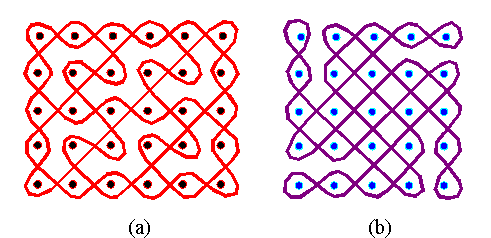Figure 1: Examples - (a) Tchokwe sand drawing, (b) Tamil threshold design. When analysing sand drawings from the Tchokwe (Angola) [Gerdes, 1993-94] and threshold designs from the Tamil (South India) [Gerdes, 1989; 1993-94, chap. 11; 1995], I found that several of them (see the two examples in Figure 1) may be generated in the following way.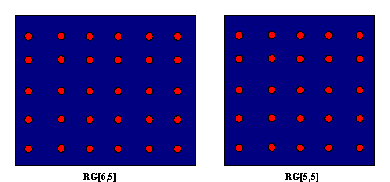Figure 2: RG[6,5] and RG[5,5]. Consider a rectangular grid RG[m,n] with vertices (0,0),(2m,0), (2m,2n), and (0,2n) and having as points (2s-1, 2t-1), where s = 1,...,m, and t = 1,...,n, and m and n two arbitrary natural numbers. Figure 2 displays RG[6,5] and RG[5,5]. A curve like that shown in Figure 1 is the smooth version of a closed polygonal path described by a lightray emitted from the point (1,0), making an angle of 45 degrees with the sides of the rectangular grid RG[m,n] (see the example in Figure 3).Figure 3: Emission of a light ray from the point (0,1).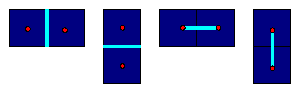Figure 4: Possible positions of mirrors.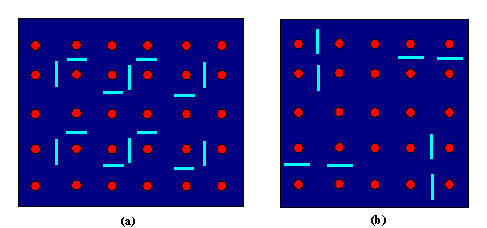Figure 5: Mirror designs that generate the curves of Figure 1. Both curves are rectangle-filling in the sense that they 'embrace' all grid points. Such curves we will call (rectangle-filling) mirror curves. The rectangular grids together with the mirrors which generate the curves will be called mirror designs. Figure 6a displays the mirror design that leads to the Celtic knot design in Figure 6b (Gerdes, 1993-94, chap. 12).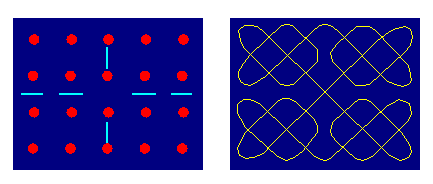Figure 6: Example of a Celtic knot design as a mirror curve. Properties and classes of mirror curves are analysed by Gerdes (1990, 1993-94, chap. 4-8) and by Jablan (1995), who also establishes links with the theory of cellular automata, Polya's enumeration theory, combinatorial geometry, topology, and knot theory.